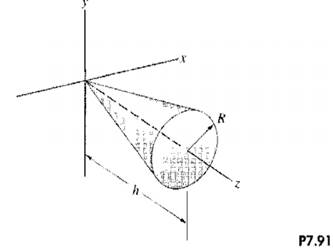### Create an Account

Home / Questions / Determine the mass moments of inertia of the homogeneous cone in problem 791 about the x a...

# Determine the mass moments of inertia of the homogeneous cone in problem 791 about the x and y axes and compare your results with the values given in Appendix C problem 791 The homogeneous cone

Determine the mass moments of inertia of the homogeneous cone in problem 7.91 about the x and y axes and compare your results with the values given in Appendix C.

problem 7.91

The homogeneous cone is of mass m. Determine its mass moment of inertia about the z axis and compare your result with the value given in Appendix C.

Strategy: Use the same approach we used in obtain the moments of inertia of a homogeneous cylinder.Jun 15 2020 View more View LessSubscribe To Get Solution